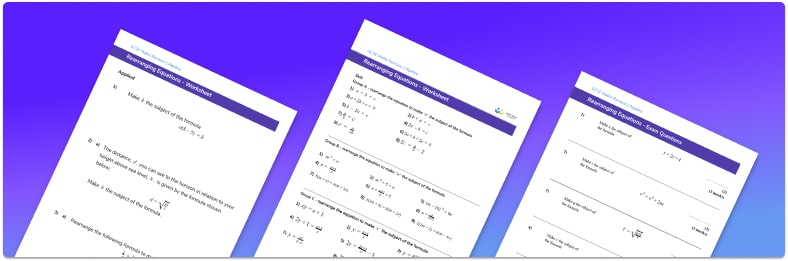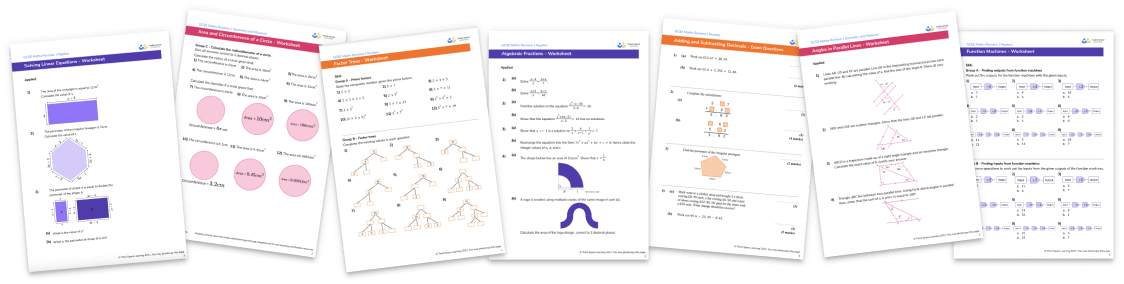# Rearranging Equations Worksheet• Section 1 of the  rearranging equations worksheet contains 27 skills-based rearranging equations questions, in 3 groups to support differentiation
• Section 2 contains 3 applied questions with a mix of worded problems and deeper problem solving questions
• Section 3 contains 7 foundation and higher level GCSE exam questions
• Answers and a mark scheme for all keyword questions are provided
• Questions follow variation theory with plenty of opportunities for students to work independently at their own level
• All questions in the printable worksheet created by fully qualified expert secondary maths teachers
• Suitable for GCSE maths revision for AQA, OCR and Edexcel exam boards

• This field is for validation purposes and should be left unchanged.

You can unsubscribe at any time (each email we send will contain an easy way to unsubscribe). To find out more about how we use your data, see our privacy policy.

### Rearranging equations at a glance

Rearranging equations manipulates the variables and constant terms in order to change the subject of the equation.

A common way to rearrange an equation is to make x the subject. This involves using inverse operations in a series of steps to leave x on one side of the equation (usually the left hand side), and all the other terms on the other side of the equals sign. Rearranging may involve addition, subtraction, multiplication and division along with operations such as square and square root.

Algebraic manipulation is sometimes required to simplify algebraic expressions before rearranging an equation such as collecting like terms, expanding brackets or factorising. Simple equations may require just one or two steps to rearrange however sometimes more steps may be required.

The skills used in rearranging algebraic equations can also be applied to rearranging formulae to allow us to make a particular unknown variable the subject of a formula. This is useful when applying mathematical formulae such as the formula for the circumference of a circle, or the speed formula. It is also useful when changing the subject of the formulas in science such as suvat.

Looking forward, students can progress with more rearranging equations worksheets to additional algebra worksheets, for example a simplifying expressions worksheetFor more teaching and learning support on Algebra our GCSE maths lessons provide step by step support for all GCSE maths concepts.

## Do you have KS4 students who need more focused attention to succeed at GCSE?There will be students in your class who require individual attention to help them succeed in their maths GCSEs. In a class of 30, it’s not always easy to provide.

Help your students feel confident with exam-style questions and the strategies they’ll need to answer them correctly with our dedicated GCSE maths revision programme.

Lessons are selected to provide support where each student needs it most, and specially-trained GCSE maths tutors adapt the pitch and pace of each lesson. This ensures a personalised revision programme that raises grades and boosts confidence.

Find out more